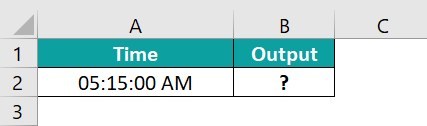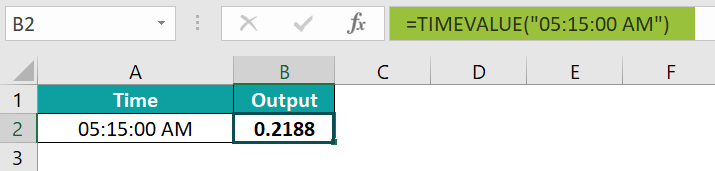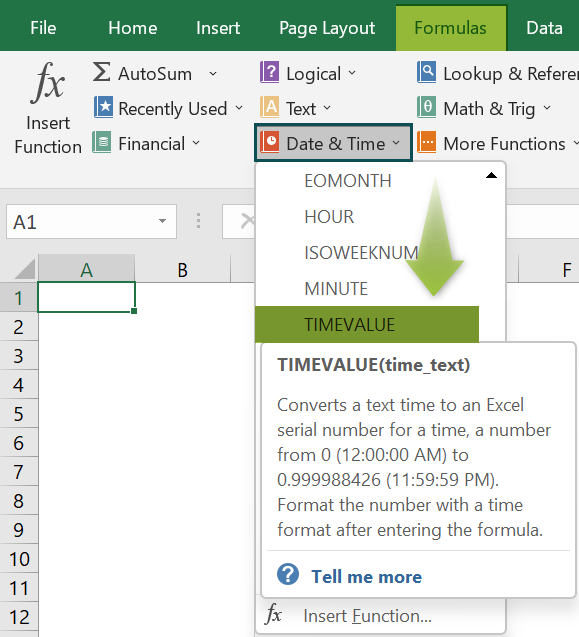# Timevalue Excel FunctionArticle byExcelMojo Team## What Is Timevalue Function In Excel?

The TIMEVALUE Function in Excel converts a text representation of a time into an Excel-recognized time format. The result will be a decimal value that resets to 0 every 24 hours. Excel only recognizes time values stored as numeric values. The TIMEVALUE Excel function is a “Date & Time” inbuilt function, so we can insert the formula from the “Function Library” or enter it directly in the worksheet.

For example, we will calculate the time value for the following table using the TIMEVALUE Excel function.

Select cell B2, enter the formula =TIMEVALUE (“2:10:01 AM”), and press “Enter”. The result is ‘0.09029’, as shown below.

###### Key Takeaways
• The TIMEVALUE function returns the decimal number of the time represented by a text string, and also returns a real-time time value.
• If the “time_text” argument value is a combination of time and date, such as (“02-12-2022 09:20:50”), then the function returns only the time value ignoring the date value.
• The output of the TIMEVALUE function resets to 0 every 24 hours.
• Since there is only one argument in the function, we can enter the cell value directly into the formula but within double quotes.

### TIMEVALUE() Excel Formula

The syntax of the TIMEVALUE() Excel Formula is,

The argument of the TIMEVALUE() Excel Formula is,

• time_text: It is a mandatory argument. It is a text string representing time in any time format.

### How To Use TIMEVALUE Excel Function?

We can use the TIMEVALUE Excel function in 2 ways, namely,

1. Access from the Excel ribbon.
2. Enter in the worksheet manually.

#### Method #1 – Access from the Excel ribbon

Choose an empty cell for the output → select the “Formulas” tab → go to the “Function Library” group → click the “Date & Time” option drop-down → select the “TIMEVALUE” function, as shown below.

The “Function Arguments” window appears. Enter the argument value in the “Time_text” field → click “OK”, as shown below.

#### Method #2 – Enter in the worksheet manually

1. Select an empty cell for the output.
2. Type =TIMEVALUE( in the selected cell. [Alternatively, type =T and double-click the TIMEVALUE function from the list of suggestions shown by Excel.]
3. Enter the argument as cell value or cell reference in Excel and close the brackets.
4. Press the “Enter” key.

We will calculate the time value using TIMEVALUE Excel function for the time in different formats.

In the table, the data is,

• Column A contains the Date.
• Column B displays the Output.

The procedure to evaluate the dates using TIMEVALUE in Excel is,

Enter the formulas as follows:

• =TIMEVALUE(“10:50:00 AM”) in cell B2,
• =TIMEVALUE(“2:10 PM”) in cell B3,
• =TIMEVALUE(“01:20:30”) in cell B4, and
• =TIMEVALUE(“05:40”) in cell B5.
• Press “Enter” after each formula.

The results are shown above. Column C is for our reference.

### Examples

We will understand some advanced scenarios using the TIMEVALUE Excel function examples.

#### Example #1

We will calculate the time value by ignoring the date using the TIMEVALUE function.

In the table, the data is,

• Column A contains the Value.
• Column B displays the Output.

The procedure to evaluate the dates by the TIMEVALUE Function in Excel is,
Select cell B2, enter the formula =TIMEVALUE(“02-12-2022 09:20:50”), and press the “Enter” key.

The result is “0.38947”, as shown above.

#### Example #2

We will calculate the #VALUE! error using the TIMEVALUE function.

In the table, the data is,

• Column A contains the Time.
• Column B displays the Output.

The procedure to evaluate the dates by the TIMEVALUE Function in Excel is,
Select cell B2, enter the formula =TIMEVALUE(“A2”), and press the “Enter” key.

The result is a “#VALUE!” error, as shown above, because we cannot use the cell reference with or without double quotes.

#### Example #3

We will calculate the time value using the TIMEVALUE function and the CONCAT() function for the time given in different cells.

In the table, the data is,

• Column A contains the Time.
• Column B contains the Minute.
• Column C contains the Second.
• Column D displays the Output.

The procedure to evaluate the dates by the TIMEVALUE Function in Excel is,

Select cell B2, enter the formula =TIMEVALUE(CONCAT(A2,”:”,B2,”:”,C2)), i.e., enter the value of ‘time_text’ as A2 “:” B2 “:” C2, and press the “Enter” key.

The result is “0.1755”, as shown above.

### Important Things To Note

• The supplied value must be valid, i.e., “1:10:12 AM” or “2:1:10”.
• The #VALUE! error occurs,
• If the argument’s value cannot be recognized as a valid Excel time.
• When the cell reference is entered instead of the cell value, with or without double quotes.
• If a text string is entered as the argument value within double quotes.
• When a text string is entered as the argument value directly without double quotes, we get the #NAME?” error.

1. What does the TIMEVALUE function do in Excel?

The TIMEVALUE Function in Excel converts the time values, which are text strings, into an Excel time. Excel only recognizes time values stored as numeric values.

The syntax of the TIMEVALUE function is =TIMEVALUE (time_text).

2. What is the difference between TIMEVALUE and DATEVALUE functions in Excel?

The TIMEVALUE function checks the cell values or the input text strings, and returns valid TIME values. The DATEVALUE function checks the cell values or the input text strings, and returns valid DATE values as preset in Excel.

The formula for calculating the date is “=DATEVALUE(date_text),” and the formula for calculating the time is “=TIMEVALUE (time_text)”.

3. How does the TIMEVALUE function work in Excel?

The TIMEVALUE function works by following the below steps,

1) Select an empty cell for the output.
2) Type =TIMEVALUE( in the selected cell. [Alternatively, type =T and double-click the TIMEVALUE function from the list of suggestions shown by Excel.]
3) Enter the argument as cell value or cell reference and close the brackets.
4) Press the “Enter” key.

For example, we will calculate the time value using the TIMEVALUE Excel function.
In the table, the data is,
Column A contains the Time.
Column B displays the Output.The procedure to evaluate the dates by the TIMEVALUE Function is,

Select cell B2, enter the formula = TIMEVALUE(“05:15:00 AM”), and press the “Enter” key.The result is “0.2188”, as shown above.

4. Where is the TIMEVALUE Function in Excel?

The TIMEVALUE Function in Excel is inserted using the following steps:

Choose an empty cell for the output → select the “Formulas” tab → go to the “Function Library” group → click the “Date & Time” option drop-down → select the “TIMEVALUE” function, as shown below.